# Algebra 3/4 : Properties of Logarithms & Exponents

## Example Questions

### Example Question #1 : Properties Of Logarithms & Exponents

Convert the expression from logarithmic to exponential.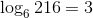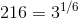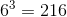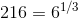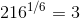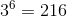Explanation:

To convert the logarithmic expression to exponential form, recall the change of base formula.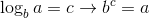Apply the change of base formula to this particular logarithmic expression.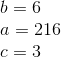The exponential form becomes### Example Question #2 : Properties Of Logarithms & Exponents

Convert the expression from logarithmic to exponential.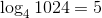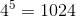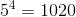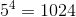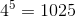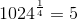Explanation:

To convert the logarithmic expression to exponential form, recall the change of base formula.Apply the change of base formula to this particular logarithmic expression.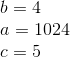The exponential form becomes### Example Question #3 : Properties Of Logarithms & Exponents

Convert the expression from logarithmic to exponential.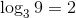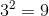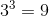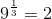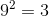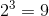Explanation:

To convert the logarithmic expression to exponential form, recall the change of base formula.Apply the change of base formula to this particular logarithmic expression.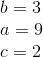The exponential form becomes### Example Question #4 : Properties Of Logarithms & Exponents

Convert the expression from logarithmic to exponential.Explanation:

To convert the logarithmic expression to exponential form, recall the change of base formula.Apply the change of base formula to this particular logarithmic expression.The exponential form becomes### Example Question #5 : Properties Of Logarithms & Exponents

Convert the expression from logarithmic to exponential.Explanation:

To convert the logarithmic expression to exponential form, recall the change of base formula.Apply the change of base formula to this particular logarithmic expression.The exponential form becomes### All Algebra 3/4 Resources